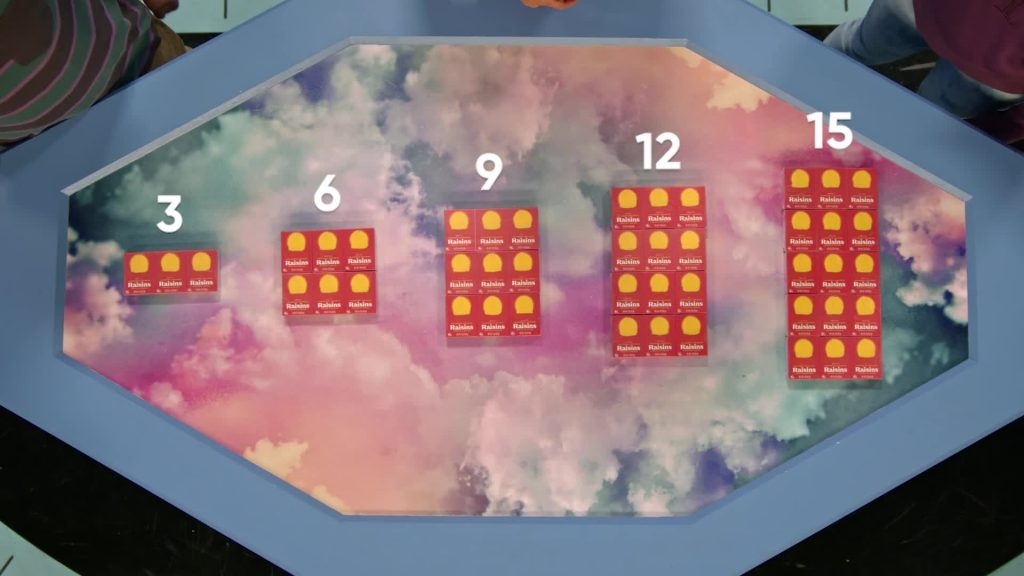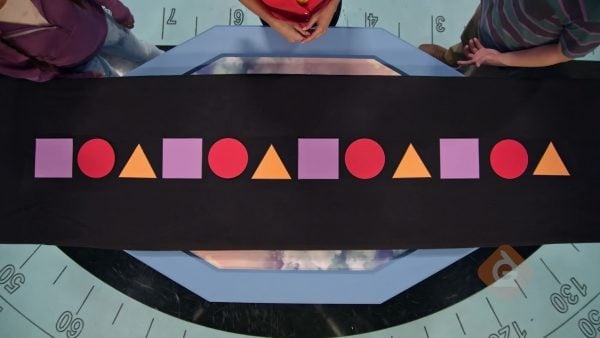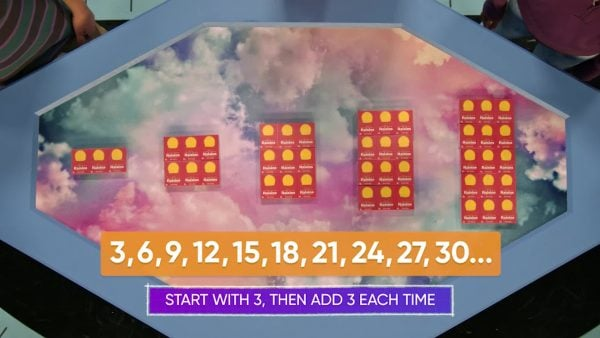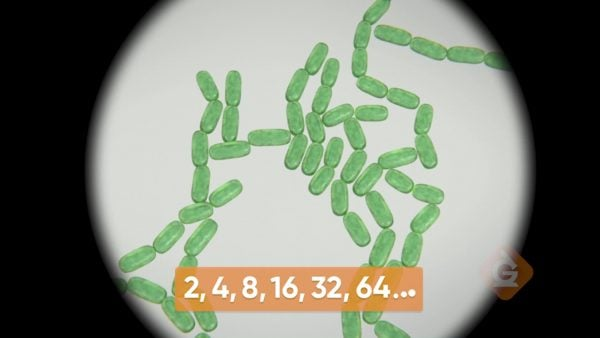Read how to Generate A Pattern of Shapes or Numbers | Grades 3, 4, & 5
1%
It was processed successfully!WHAT DOES IT MEAN TO GENERATE A PATTERN OF NUMBERS?

A pattern is a set of shapes or numbers that change based on a rule. A pattern could be repeating, like ABABAB, or continue on, like ABCDEFG.

To better understand generating a pattern of shapes or numbers…

WHAT DOES IT MEAN TO GENERATE A PATTERN OF NUMBERS?. A pattern is a set of shapes or numbers that change based on a rule. A pattern could be repeating, like ABABAB, or continue on, like ABCDEFG. To better understand generating a pattern of shapes or numbers…

## LET’S BREAK IT DOWN!

### Shape PatternsApril's bracelet is made of parts that are circles and triangles. The bracelet has a pattern: circle, triangle, circle, triangle, … This is an example of a repeating pattern. Since the shapes take turns being next, you can predict that the next shape is another circle! A repeating pattern uses a rule that repeats over and over. A pattern is something that repeats in a predictable way. The slightly more challenging pattern could be: circle, circle, pentagon, circle, circle, pentagon, circle, circle, pentagon. In this example, the rule is you have two circles, and then a pentagon. Then that pattern of three shapes repeats. You can predict the next shapes, two circles and a pentagon! Another pattern is: triangle, square, pentagon, hexagon. This one is harder because none of the shapes are repeating. Think about the properties of these shapes. Each shape has one more side than the shape before it! Hexagons have 6 sides, so the next shape would have 7 sides, a heptagon. Try this one yourself: Draw a new shape pattern. Trade patterns with a partner. Identify your partner's pattern.

Shape Patterns April's bracelet is made of parts that are circles and triangles. The bracelet has a pattern: circle, triangle, circle, triangle, … This is an example of a repeating pattern. Since the shapes take turns being next, you can predict that the next shape is another circle! A repeating pattern uses a rule that repeats over and over. A pattern is something that repeats in a predictable way. The slightly more challenging pattern could be: circle, circle, pentagon, circle, circle, pentagon, circle, circle, pentagon. In this example, the rule is you have two circles, and then a pentagon. Then that pattern of three shapes repeats. You can predict the next shapes, two circles and a pentagon! Another pattern is: triangle, square, pentagon, hexagon. This one is harder because none of the shapes are repeating. Think about the properties of these shapes. Each shape has one more side than the shape before it! Hexagons have 6 sides, so the next shape would have 7 sides, a heptagon. Try this one yourself: Draw a new shape pattern. Trade patterns with a partner. Identify your partner's pattern.Lists of numbers can also show patterns, not just shapes. Let's say a dish has 3 raisins. The next dish has 6 raisins. Then there are 9, 12, 15, and 18 raisins in the next dishes. What pattern can you find? The number of raisins in each dish increases by 3. You add 3 to get the next number. 18 + 3 = 21. So the next dish has 21 raisins. Continue the pattern to get 24, 27, and 30 raisins. It’s kind of like predicting the future! There is a second pattern you can also use here: 3 is odd, 6 is even, 9 is odd, 12 is even, and so on. That is because an even number plus an odd number is an odd number, and an odd number plus an odd number is an even number. So, the pattern goes back and forth between odd and even. Here is another example. Sports drinks come in packs of 6. What pattern do six of these packs form? The total number of sports drinks is: 6, 12, 18, 24, 30, 36. The rule is: add 6 for each new pack of drinks. These numbers are all even, because we started with 6 (an even number) and we add 6 (an even number) each time. That is, adding two even numbers results in another even number. The numbers in this list are all twice as much as the numbers in the raisins list. One dish had 3 raisins, and we added threes. One pack has 6 sports drinks, and we add sixes. Try this one yourself: Write three new number patterns: one where the numbers are all even, one where some numbers are even and others are odd, and one where the numbers are all odd.

Addition Examples Lists of numbers can also show patterns, not just shapes. Let's say a dish has 3 raisins. The next dish has 6 raisins. Then there are 9, 12, 15, and 18 raisins in the next dishes. What pattern can you find? The number of raisins in each dish increases by 3. You add 3 to get the next number. 18 + 3 = 21. So the next dish has 21 raisins. Continue the pattern to get 24, 27, and 30 raisins. It’s kind of like predicting the future! There is a second pattern you can also use here: 3 is odd, 6 is even, 9 is odd, 12 is even, and so on. That is because an even number plus an odd number is an odd number, and an odd number plus an odd number is an even number. So, the pattern goes back and forth between odd and even. Here is another example. Sports drinks come in packs of 6. What pattern do six of these packs form? The total number of sports drinks is: 6, 12, 18, 24, 30, 36. The rule is: add 6 for each new pack of drinks. These numbers are all even, because we started with 6 (an even number) and we add 6 (an even number) each time. That is, adding two even numbers results in another even number. The numbers in this list are all twice as much as the numbers in the raisins list. One dish had 3 raisins, and we added threes. One pack has 6 sports drinks, and we add sixes. Try this one yourself: Write three new number patterns: one where the numbers are all even, one where some numbers are even and others are odd, and one where the numbers are all odd.

### Fuel GaugeSome patterns have numbers that slowly go down, like the fuel gauge on a car’s dashboard. One car's gauge starts at 20 liters in the gas tank, then goes down to 18, 16, 14, 12, 10, and 8. This makes sense because you use gas as you drive, so there’s less and less gas left in the tank. This is an example of a decreasing number pattern, where each new number is less than the previous one. In this case, the rule is subtract 2. This pattern continues until the gauge shows 0, which means there’s no more gas left in the tank. Try this one yourself: Think of a new real-world situation that has a decreasing number pattern. Does the pattern end or go on forever? Try to convince someone else that you’re correct.

Fuel Gauge Some patterns have numbers that slowly go down, like the fuel gauge on a car’s dashboard. One car's gauge starts at 20 liters in the gas tank, then goes down to 18, 16, 14, 12, 10, and 8. This makes sense because you use gas as you drive, so there’s less and less gas left in the tank. This is an example of a decreasing number pattern, where each new number is less than the previous one. In this case, the rule is subtract 2. This pattern continues until the gauge shows 0, which means there’s no more gas left in the tank. Try this one yourself: Think of a new real-world situation that has a decreasing number pattern. Does the pattern end or go on forever? Try to convince someone else that you’re correct.

### BacteriaScientists use number patterns to study bacteria and how it grows. After only 10 minutes, 2 bacteria split into 4. After 10 more minutes, the 4 split into 8. 10 minutes later, they split to 16! Then there are 32, and eventually 64 bacteria. In this instance, addition just doesn’t work. There’s a new kind of rule: multiply by 2. You can predict that the number of bacteria in another 10 minutes is 64 × 2 = 128. These are all even numbers, because multiplying an even number by an even number always gives you an even number. A pattern that’s related to this, but a little different, is: 243, 81, 27, 9, 3. This is a decreasing pattern, but subtraction doesn’t do the trick. Notice that 3 × 3 = 9, 9 × 3 = 27, 27 × 3 = 81, and 81 × 3 =243. The rule must be the opposite of multiplying by 3: dividing by 3! The next number is 1. Try this one yourself: Write a multiplication pattern and a division pattern. Trade patterns with a partner. Figure out the next three numbers of your partner's pattern.

Bacteria Scientists use number patterns to study bacteria and how it grows. After only 10 minutes, 2 bacteria split into 4. After 10 more minutes, the 4 split into 8. 10 minutes later, they split to 16! Then there are 32, and eventually 64 bacteria. In this instance, addition just doesn’t work. There’s a new kind of rule: multiply by 2. You can predict that the number of bacteria in another 10 minutes is 64 × 2 = 128. These are all even numbers, because multiplying an even number by an even number always gives you an even number. A pattern that’s related to this, but a little different, is: 243, 81, 27, 9, 3. This is a decreasing pattern, but subtraction doesn’t do the trick. Notice that 3 × 3 = 9, 9 × 3 = 27, 27 × 3 = 81, and 81 × 3 =243. The rule must be the opposite of multiplying by 3: dividing by 3! The next number is 1. Try this one yourself: Write a multiplication pattern and a division pattern. Trade patterns with a partner. Figure out the next three numbers of your partner's pattern.

## GENERATE NUMBER PATTERNS (SEQUENCES THAT FOLLOW A GIVEN RULE) VOCABULARY

Sequence
Shapes or numbers in a certain order.
Pattern
Numbers, objects, or shapes arranged following a rule or rules.
Repeating pattern
A rule that gets used over and over.
Increasing pattern
A pattern where each new number is more than the previous one.
Decreasing pattern
A pattern where each new number is less than the previous one.
2D Shape
Flat shapes made by straight or curved lines. Some examples of 2D shapes are circles, squares, rectangles, and triangles.
one of the line segments that make a 2D shape. A triangle has 3 sides.

## GENERATE NUMBER PATTERNS (SEQUENCES THAT FOLLOW A GIVEN RULE) DISCUSSION QUESTIONS

### What advice would you give a student who was absent today about how to figure out shape patterns?

Look for descriptions of the direction an object points, where the curves and line segments are, where new pieces are, or for missing or empty parts of a figure.

### Give an example of an “increasing” and a “decreasing” number pattern. Explain the rule for each pattern.

Increasing by 5s: 5, 10, 15, 20; decreasing by 5s: 20, 15, 10, 5.

### Give examples of a rule that makes a multiplication pattern and a rule that makes a division pattern. Explain the rule for each pattern.

Multiply by three: 1, 3, 9, 27, 81; divide by ten: 1,000, 100, 10, 1.

### How is the rule “double” different from the rule “double plus 10”?

The second rule has two steps. If you double 3 you get 6, but if you double 3 and add 10 you get 16.

### Give an example of a rule that goes on forever, and a rule that doesn’t.

"Multiply by 2” goes on forever; a fuel gauge goes down as a car drives, and stops when the gas tank is empty.
X

## Success

We’ve sent you an email with instructions how to reset your password.
Ok